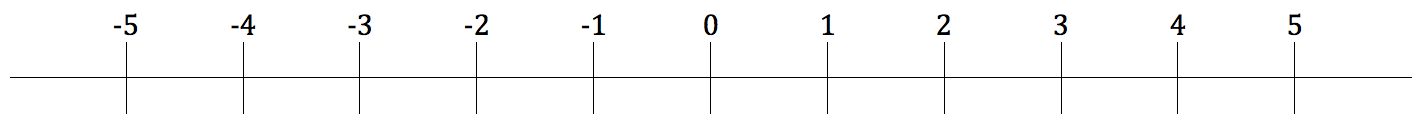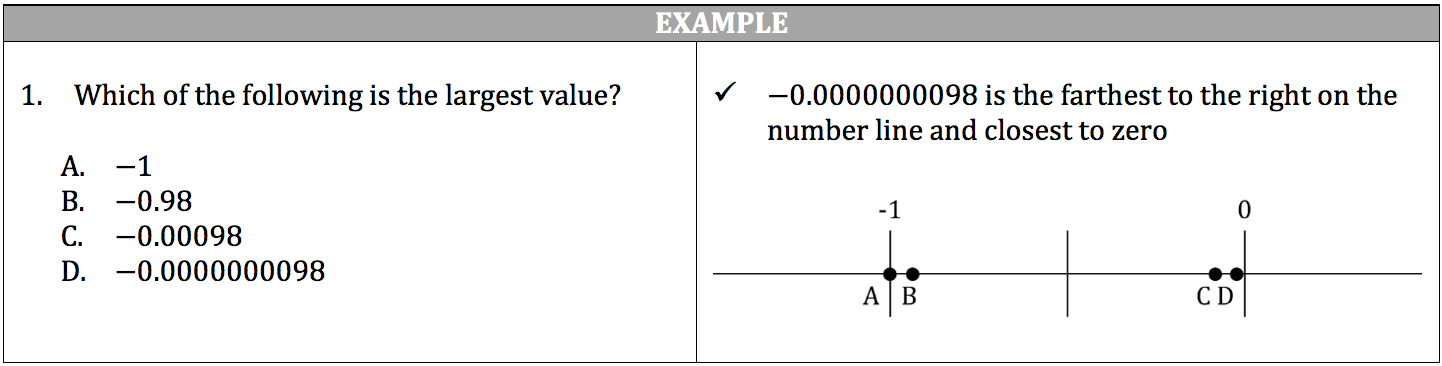Number lines are used to visually represent the relationships between integers, fractions, and decimals and to show how mathematic operations work in these relationships. Some number lines are shown with arrows pointing in opposite directions on either end of the line to designate a continuity of sequence in positive and negative numbers.

• The number line is shown as a line with equally spaced tick marks along the whole line.
• Numbers to the left of zero are negative (‐ sign always shown) and numbers to the right of zero are positive (+ sign usually not shown).• If you move to the right on the number line, the numbers always increase in size.
• If you move to the left on the number line, the numbers always decrease in size.

Unless specifically noted, number lines are generally drawn to scale, which will enable you to make accurate estimations of distances and positions of points on a number line. When you compare relationships between negative numbers, remember that the number closest to zero is the largest number.